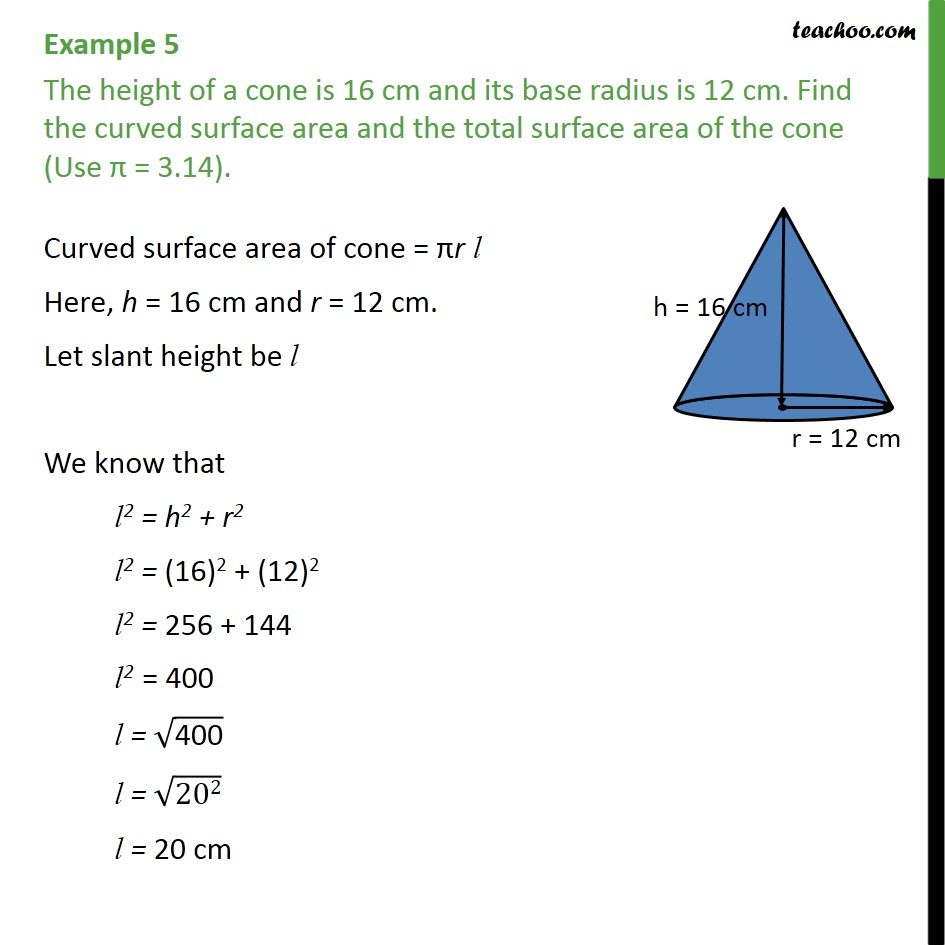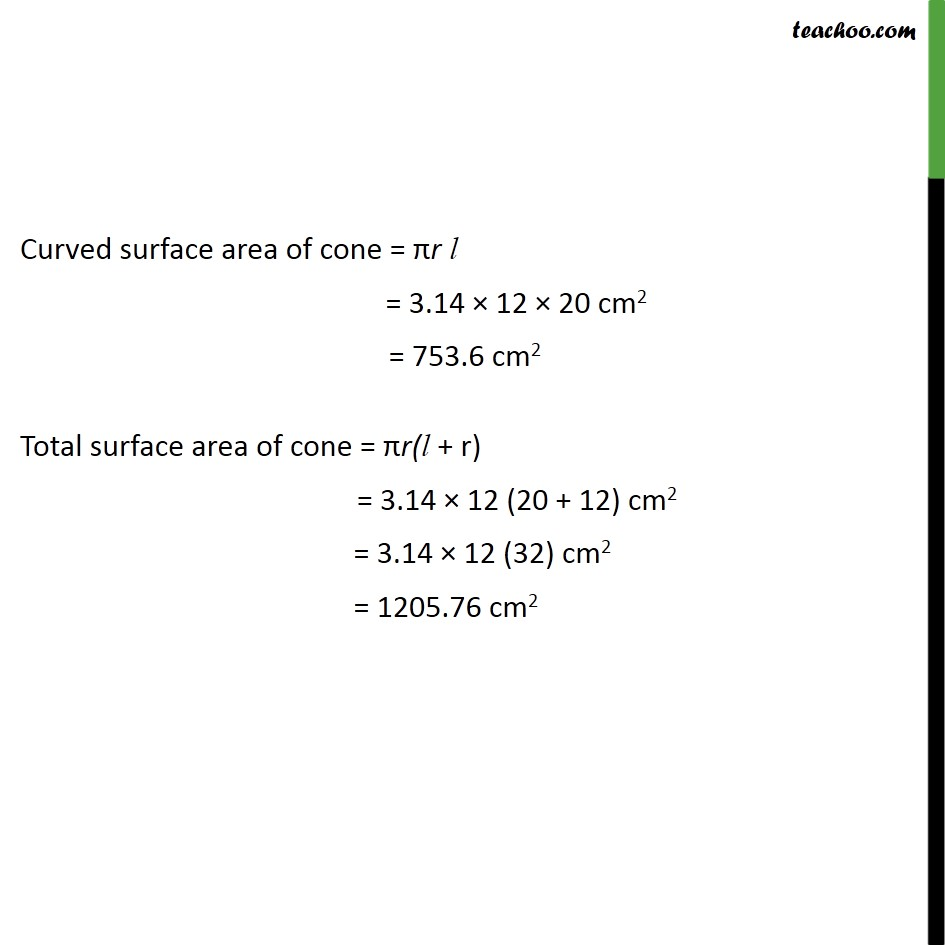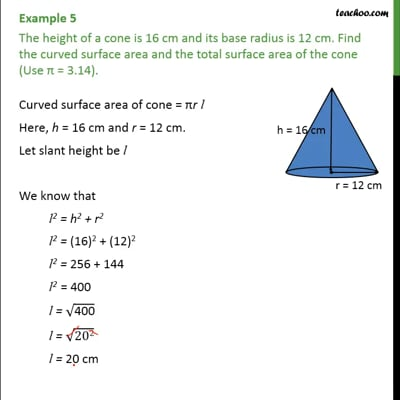Examples

Chapter 13 Class 9 Surface Areas and Volumes (Term 2)
Serial order wiseThis video is only available for Teachoo black users

### Transcript

Example 5 The height of a cone is 16 cm and its base radius is 12 cm. Find the curved surface area and the total surface area of the cone (Use π = 3.14). Curved surface area of cone = πr l Here, h = 16 cm and r = 12 cm. Let slant height be l We know that l2 = h2 + r2 l2 = "(16)2 + (12)2" l2 = "256 + 144" l2 = 400 l = √("400" ) l = √("202" ) l = 20 cm Curved surface area of cone = πr l = 3.14 × 12 × 20 cm2 = 753.6 cm2 Total surface area of cone = πr(l + r) = 3.14 × 12 (20 + 12) cm2 = 3.14 × 12 (32) cm2 = 1205.76 cm2